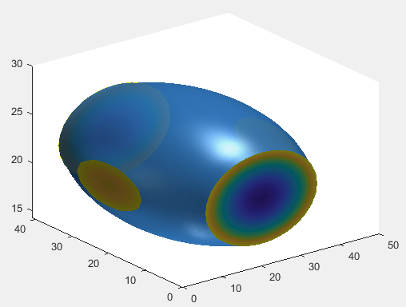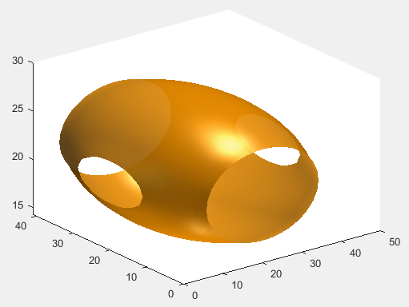## Managing Properties of Chart Classes

When you develop a custom chart as a subclass of the `ChartContainer` base class, you can use certain techniques to make your code more robust, efficient, and tailored to the needs of your users. These techniques focus on how you define and manage the properties of your class. Use any that are helpful for the type of visualization you want to create and the user experience you want to provide.

• Initialize property values — Set the default state of the chart in case your users call the implicit constructor without any input arguments.

• Validate property values — Ensure that the values are valid before using them to perform a calculation or configure one of the underlying graphics objects in your chart.

• Customize the property display — Provide a customized list of properties when a user references the chart object without semicolon.

• Optimize the `update` method — Improve the performance of the `update` method when only a subset of your properties are used in a time-consuming calculation.

### Initialize Property Values

Assign default values for all of the public properties of your class. Doing so configures a valid chart if the user omits some of the name-value pair arguments when they call the constructor method.

For properties that store coordinate data, set the initial values to `NaN` values or empty arrays so that the default chart is empty when the user does not specify the coordinates. Choose the default coordinates according to the requirements of the plotting functions you plan to call in your class methods. To learn about the requirements, see the documentation for the plotting functions you plan to use.

### Validate Property Values

A good practice is to verify the values of your class properties before your code uses those values. A convenient way to do this is to validate the size and class of the properties as you define them. For example, this property block validates the size and class of four properties.

```properties IsoValue (1,1) double = 0.5 Enclose {mustBeMember(Enclose,{'above','below'})} = 'below' CapVisible (1,1) matlab.lang.OnOffSwitchState = 'on' Color (1,3) double {mustBeGreaterThanOrEqual(Color,0),... mustBeLessThanOrEqual(Color,1)} = [.2 .5 .8] end```

• `IsoValue` must be a 1-by-1 array of class `double`.

• `Enclose` must have a value of either `'above'` or `'below'`.

• `CapVisible` must be a 1-by-1 array of class `matlab.lang.OnOffSwitchState`.

• `Color` must be a 1-by-3 array of class `double`, where each value is in the range `[0,1]`.

You can also validate properties that store the underlying graphics objects in your chart. To determine the class name of an object, call the corresponding plotting function at the command line, and then call the `class` function to get the class name. For example, if you plan to call the `patch` function in your `setup` method, call the `patch` function at the command line with an output argument (the input arguments do not matter). Then pass the output to the `class` function to get its class name.

```x = patch(NaN,NaN,NaN); class(x)```
```ans = 'matlab.graphics.primitive.Patch'```

Use the output of the `class` function to validate the class for the corresponding property in your class. For example, each of the following properties stores a `Patch` object.

```properties (Access = private,Transient,NonCopyable) IsoPatch (1,1) matlab.graphics.primitive.Patch CapPatch (1,1) matlab.graphics.primitive.Patch end```

Occasionally, you might want to define a property that can store different shapes and classes of values. For example, if you define a property that can store a character vector, cell array of character vectors, or string array, omit the size and class validation or use a custom property validation method.

### Customize the Property Display

One of the benefits of defining your chart as a subclass of the `ChartContainer` base class is that it also inherits from the `matlab.mixin.CustomDisplay` class. Thus, you can customize the list of properties MATLAB® displays in the Command Window when you reference the chart without a semicolon. To customize the property display, overload the `getPropertyGroups` method. Within that method, you can customize which properties are listed and the order of the list. For example, consider an `IsoSurfCapChart` class that has the following public properties.

```properties IsoValue (1,1) double = 0.5 Enclose {mustBeMember(Enclose,{'above','below'})} = 'below' CapVisible (1,1) matlab.lang.OnOffSwitchState = 'on' Color (1,3) double {mustBeGreaterThanOrEqual(Color,0),... mustBeLessThanOrEqual(Color,1)} = [.2 .5 .8] end```

The following `getPropertyGroups` method specifies the scalar object property list as `Color`, `IsoValue`, `Enclose`, and `CapVisible`.

```function propgrp = getPropertyGroups(obj) if ~isscalar(obj) % List for array of objects propgrp = getPropertyGroups@matlab.mixin.CustomDisplay(obj); else % List for scalar object propList = {'Color','IsoValue','Enclose','CapVisible'}; propgrp = matlab.mixin.util.PropertyGroup(propList); end end ```

When the user references an instance of this chart without a semicolon, MATLAB displays the customized list.

`c = IsoSurfCapChart`
```c = IsoSurfCapChart with properties: Color: [0.2000 0.5000 0.8000] IsoValue: 0.5000 Enclose: 'below' CapVisible: on ```

### Optimize the `update` Method

In most cases, the `update` method of your class reconfigures all the relevant aspects of your chart that depend on the public properties. Sometimes, the reconfiguration involves an expensive calculation that is time consuming. If the calculation involves only a subset of the properties, you can design your class to execute that code only when it is necessary.

One way to optimize the `update` method is to add these components to your class:

• Define a private property called `ExpensivePropChanged` that accepts a `logical` value. This property indicates whether any of the properties used in the expensive calculation have changed.

• Write a `set` method for each property involved in the expensive calculation. Within each `set` method, set the `ExpensivePropChanged` property to `true`.

• Write a protected method that performs the expensive calculation.

• Write a conditional statement in the `update` method that checks the value of `ExpensivePropChanged`. If the value is `true`, execute the method that performs the expensive calculation.

The following code provides a simplified implementation of this design.

```classdef OptimizedChart < matlab.graphics.chartcontainer.ChartContainer properties Prop1 Prop2 end properties(Access=private,Transient,NonCopyable) ExpensivePropChanged (1,1) logical = true end methods(Access = protected) function setup(obj) % Configure chart % ... end function update( obj ) % Perform expensive computation if needed if obj.ExpensivePropChanged doExpensiveCalculation(obj); obj.ExpensivePropChanged = false; end % Update other aspects of chart % ... end function doExpensiveCalculation(obj) % Expensive code % ... end end methods function set.Prop2(obj,val) obj.Prop2 = val; obj.ExpensivePropChanged = true; end end end```
In this case, `Prop2` is involved in the expensive calculation. The `set.Prop2` method sets the value of `Prop2`, and then it sets `ExpensivePropChanged` to `true`. Thus, the next time the `update` method runs, it calls `doExpensiveCalculation` only if `ExpensivePropChanged` is `true`. Then the `update` method continues to update other aspects of the chart.

### Example: Optimized Isosurface Chart with Customized Property Display

Define an `IsoSurfCapChart` class for displaying an `isosurface` with the associated `isocaps`. Include the following features:

• Properties that use size and class validation

• A customized property display

• An optimized `update` method that recalculates the `isosurface` and `isocaps` only if one or more of the relevant properties changed

To define this class, create a program file named `IsoSurfCapChart.m` in a folder that is on the MATLAB path. Then implement the class by following the steps in the table.

StepImplementation

Derive from the `ChartContainer` base class.

`classdef IsoSurfCapChart < matlab.graphics.chartcontainer.ChartContainer`

Define the public properties using class and size validation.

• `VolumeData`, `IsoValue`, and `Color` are parameters for the `isosurface`.

• `Enclose`, `WhichCapPlane`, and `CapVisible` are parameters for the `isocaps`.

``` properties VolumeData double = rand(25,25,25) IsoValue (1,1) double = 0.5 Enclose {mustBeMember(Enclose,{'above','below'})} = 'below' WhichCapPlane {mustBeMember(WhichCapPlane,{'all','xmin',... 'xmax','ymin','ymax','zmin','zmax'})} = 'all' CapVisible (1,1) matlab.lang.OnOffSwitchState = 'on' Color (1,3) double {mustBeGreaterThanOrEqual(Color,0),... mustBeLessThanOrEqual(Color,1)} = [.2 .5 .8] end```

Define the private properties.

• `IsoPatch` and `CapPatch` store the `Patch` objects for the `isosurface` and `isocaps`.

• `SmoothData` stores a smoothed version of the volume data.

• `ExpensivePropChanged` indicates whether the update method needs to recalculate the `isosurface` and `isocaps`.

``` properties(Access = private,Transient,NonCopyable) IsoPatch (1,1) matlab.graphics.primitive.Patch CapPatch (1,1) matlab.graphics.primitive.Patch SmoothData double = []; ExpensivePropChanged (1,1) logical = true end```

Implement the `setup` method. In this case, call the `patch` function twice to create the `Patch` objects for the `isosurface` and `isocaps`. Store the objects in the corresponding properties, and configure the axes.

``` methods(Access = protected) function setup(obj) ax = getAxes(obj); % Create two Patch objects obj.IsoPatch = patch(ax,NaN,NaN,NaN, 'EdgeColor', 'none', ... 'FaceColor',[.2 .5 .8],'FaceAlpha',0.9); hold(ax,'on'); obj.CapPatch = patch(ax,NaN,NaN,NaN,'EdgeColor', 'none', ... 'FaceColor','interp'); % Configure the axes view(ax,3) camlight(ax, 'infinite'); camlight(ax,'left'); lighting(ax, 'gouraud'); hold(ax,'off'); end```

Implement the `update` method. Decide whether to call the `doExpensiveCalculation` method by testing the value of `ExpensivePropChanged`. Then continue updating the other (less expensive) aspects of the chart.

``` function update(obj) % Perform expensive computation if needed if obj.ExpensivePropChanged doExpensiveCalculation(obj); obj.ExpensivePropChanged = false; end % Update visibility of CapPatch and update color obj.CapPatch.Visible = obj.CapVisible; obj.IsoPatch.FaceColor = obj.Color; end```

Implement the `doExpensiveCalculation` method, which smooths the volume data and recalculates the faces and vertices of the `isosurface` and `isocaps`.

``` function doExpensiveCalculation(obj) % Update isosurface obj.SmoothData = smooth3(obj.VolumeData,'box',7); [F,V] = isosurface(obj.SmoothData, obj.IsoValue); set(obj.IsoPatch,'Faces',F,'Vertices',V); isonormals(obj.SmoothData,obj.IsoPatch); % Update isocaps [m,n,p] = size(obj.SmoothData); [Xc,Yc,Zc] = meshgrid(1:n,1:m,1:p); [Fc,Vc,Cc] = isocaps(Xc,Yc,Zc,obj.SmoothData,obj.IsoValue,... obj.Enclose,obj.WhichCapPlane); set(obj.CapPatch,'Faces',Fc,'Vertices',Vc,'CData',Cc); end```

Implement the `getPropertyGroups` method to customize the property display.

``` function propgrp = getPropertyGroups(obj) if ~isscalar(obj) % List for array of objects propgrp = getPropertyGroups@matlab.mixin.CustomDisplay(obj); else % List for scalar object propList = {'Color','IsoValue','Enclose','CapVisible',... 'WhichCapPlane','VolumeData'}; propgrp = matlab.mixin.util.PropertyGroup(propList); end end end```

Implement the `set` methods for each expensive property (`VolumeData`, `IsoValue`, and `Enclose`). Within each method, set the corresponding property value, and then set `ExpensivePropChanged` to `true`.

``` methods function set.VolumeData(obj,val) obj.VolumeData = val; obj.ExpensivePropChanged = true; end function set.IsoValue(obj, val) obj.IsoValue = val; obj.ExpensivePropChanged = true; end function set.Enclose(obj, val) obj.Enclose = val; obj.ExpensivePropChanged = true; end end end```

Next, create an array of volume data, and then create an instance of `IsoSurfCapChart`.

```[X,Y,Z] = meshgrid(-2:0.1:2); v = (1/9)*X.^2 + (1/16)*Y.^2 + Z.^2; c = IsoSurfCapChart('VolumeData',v,'IsoValue',0.5)```
```c = IsoSurfCapChart with properties: Color: [0.2000 0.5000 0.8000] IsoValue: 0.5000 Enclose: 'below' CapVisible: on WhichCapPlane: 'all' VolumeData: [41×41×41 double]```Change the color of `c` and hide the `isocaps`.

```c.Color = [1 0.60 0]; c.CapVisible = false;```